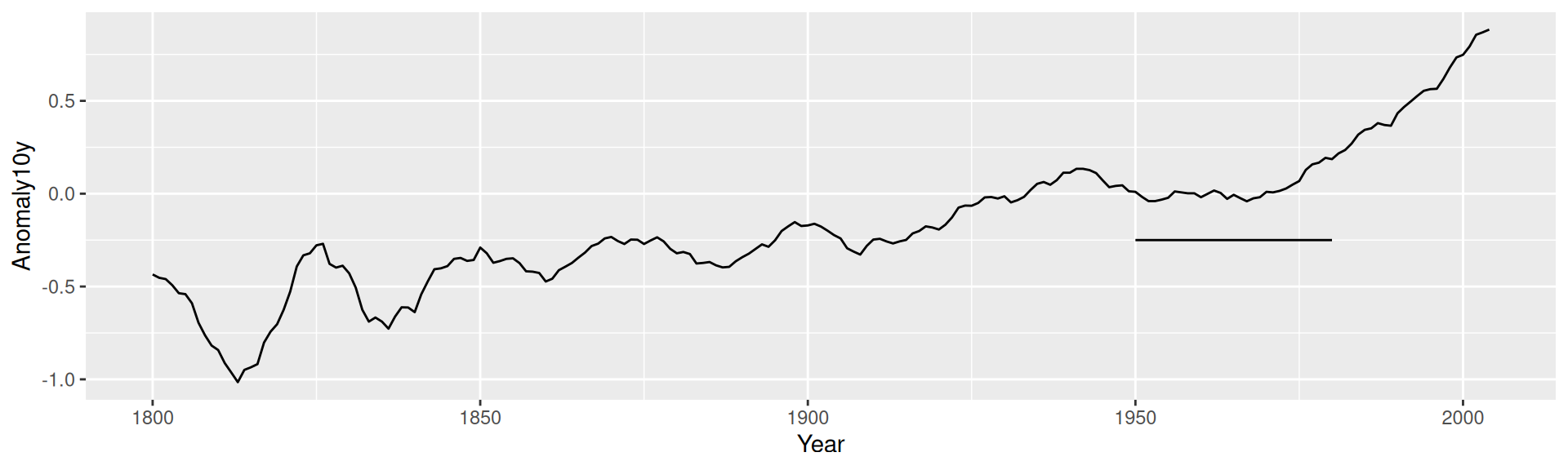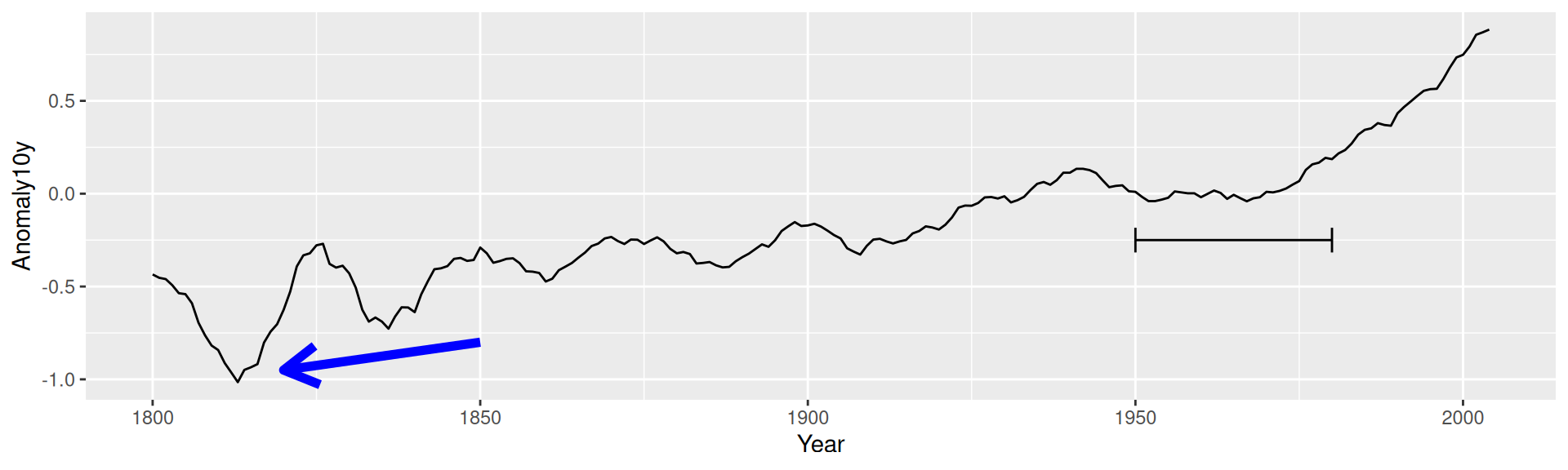## 7.4 Adding Line Segments and Arrows

### 7.4.1 Problem

You want to add line segments or arrows to a plot.

### 7.4.2 Solution

Use `annotate("segment")`. In this example, we’ll use the climate data set and use a subset of data from the Berkeley source (Figure 7.10):

``````library(gcookbook) # Load gcookbook for the climate data set

p <- ggplot(filter(climate, Source == "Berkeley"), aes(x = Year, y = Anomaly10y)) +
geom_line()

p +
annotate("segment", x = 1950, xend = 1980, y = -.25, yend = -.25)``````Figure 7.10: Line segment annotation

### 7.4.3 Discussion

It’s possible to add arrowheads or flat ends to the line segments, using `arrow()` from the grid package. In this example, we’ll do both (Figure 7.11):

``````library(grid)
p +
annotate("segment", x = 1850, xend = 1820, y = -.8, yend = -.95,
colour = "blue", size = 2, arrow = arrow()) +
annotate("segment", x = 1950, xend = 1980, y = -.25, yend = -.25,
arrow = arrow(ends = "both", angle = 90, length = unit(.2,"cm")))``````Figure 7.11: Line segments with arrow heads

The default angle is 30, and the default length of the arrowhead lines is 0.2 inches.

If one or both axes are discrete, the x and y positions are such that the categorical items have coordinate values 1, 2, 3, and so on.

For more information about the parameters for drawing arrows, load the grid package and see `?arrow`.